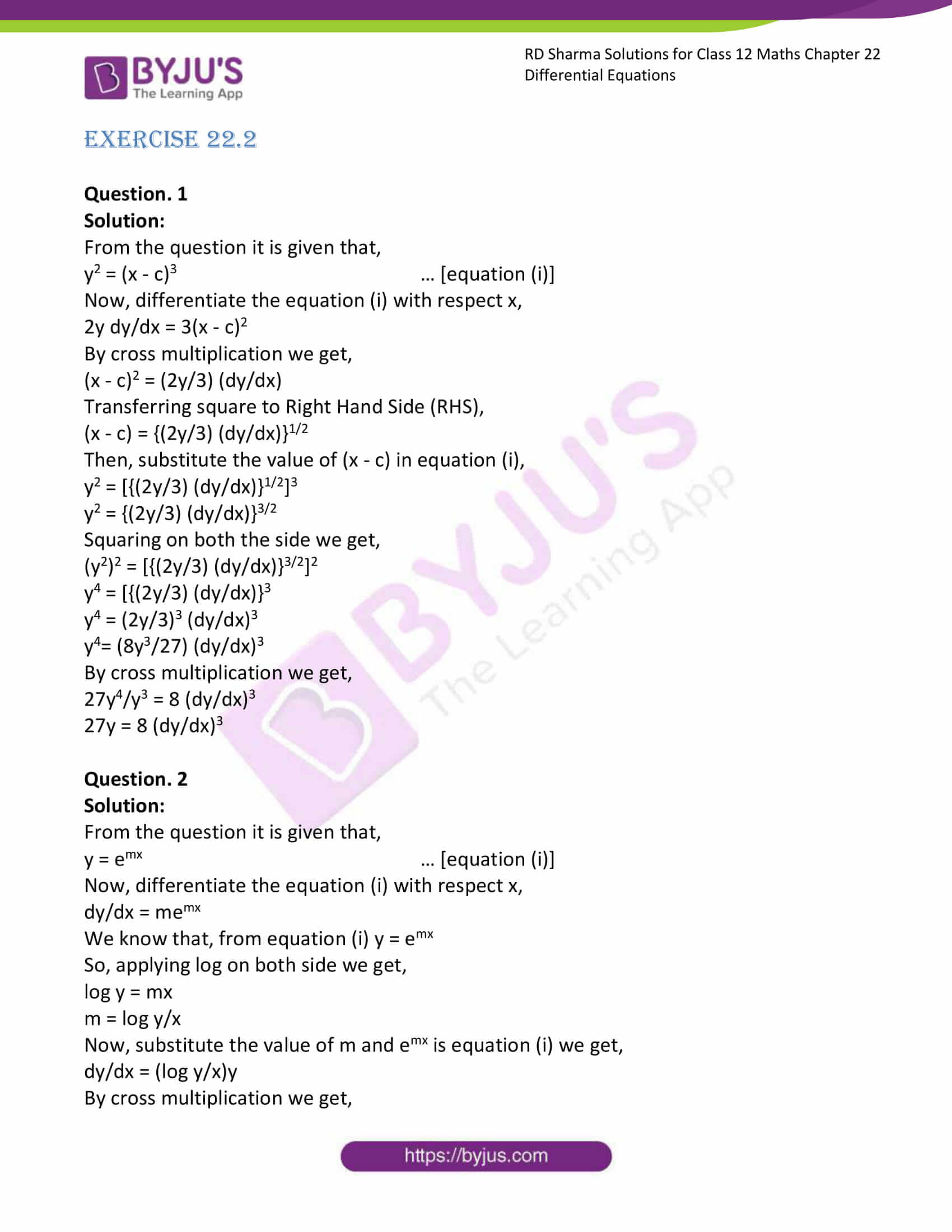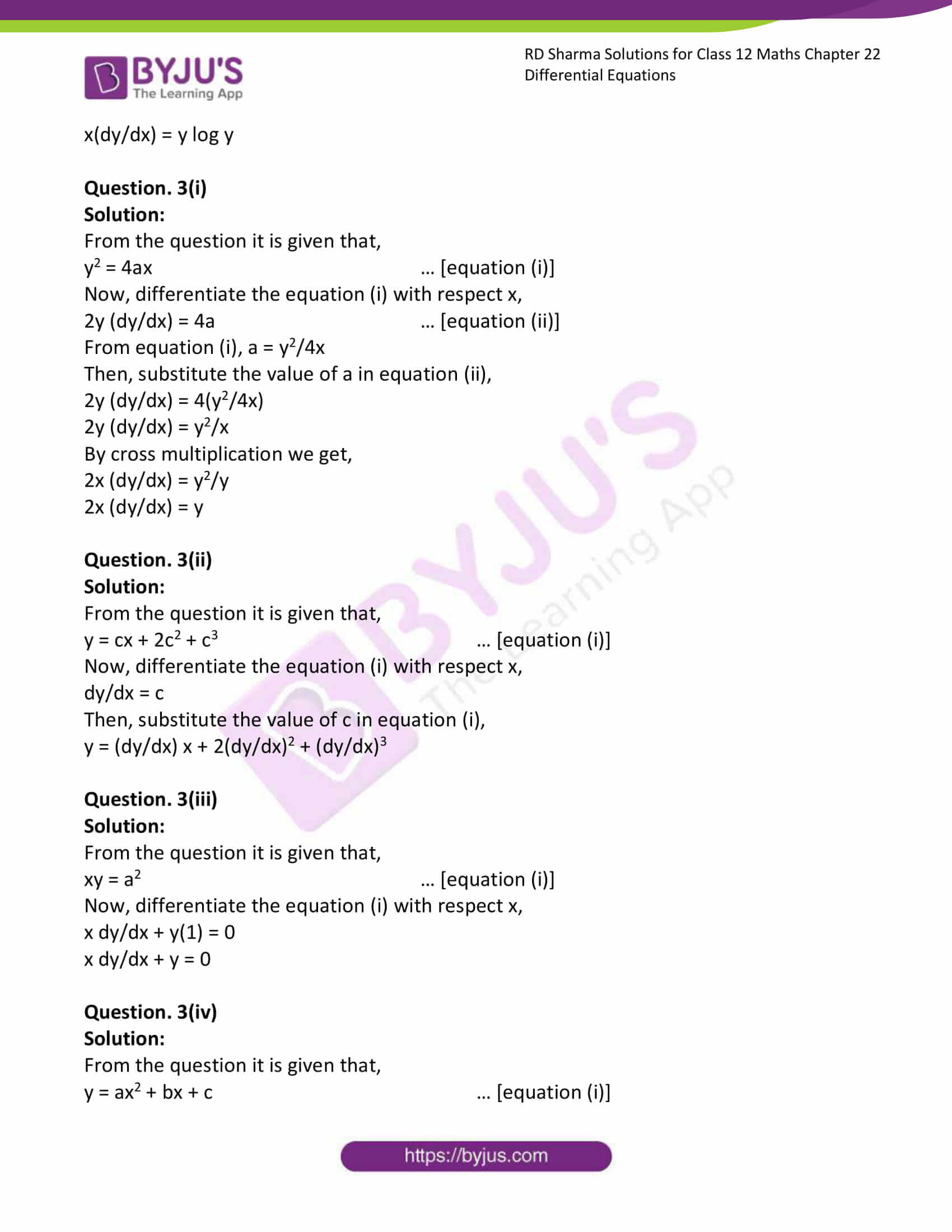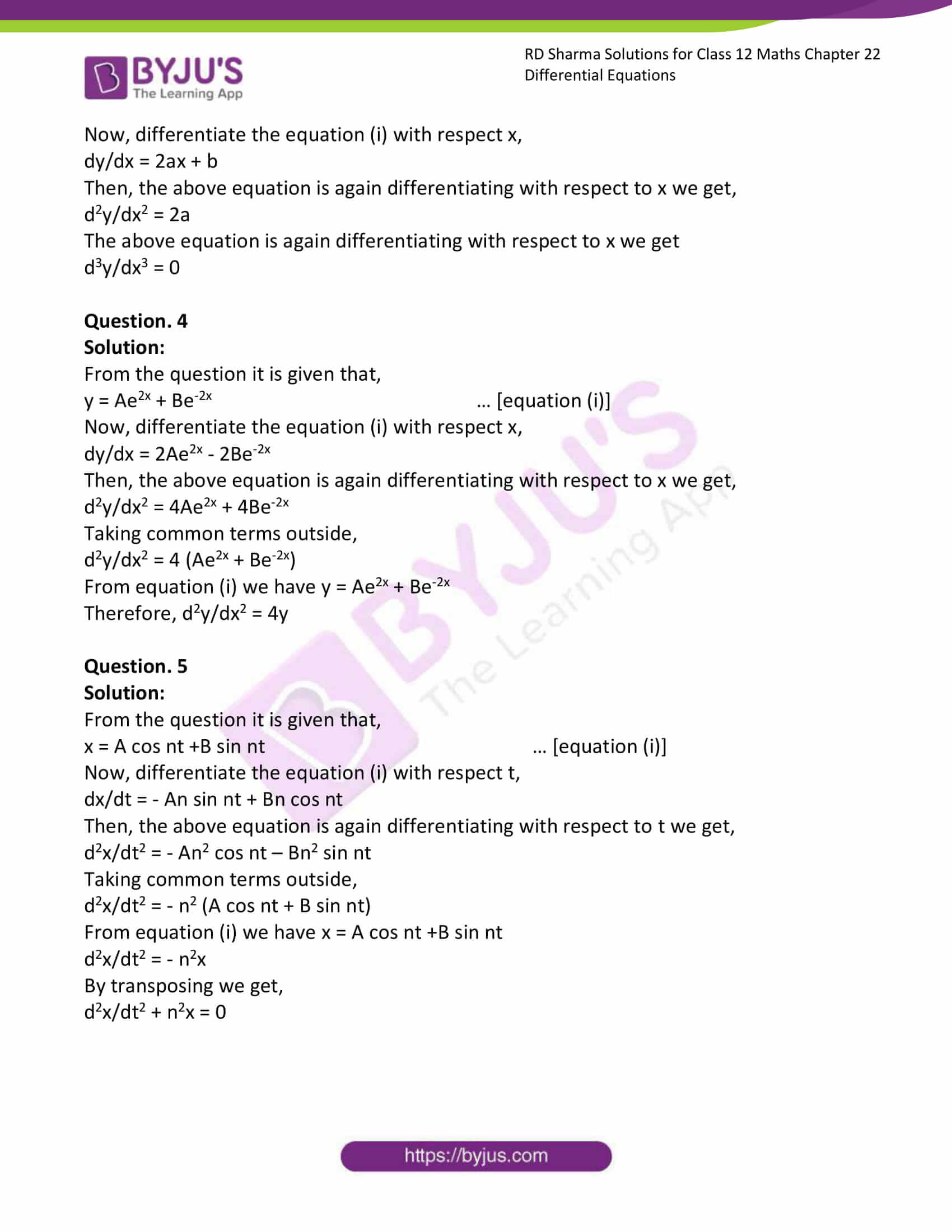# RD Sharma Solutions Class 12 Differential Equations Exercise 22.2

RD Sharma Solutions for Class 12 Maths Exercise 22.2 Chapter 22 Differential Equations are most valuable that assist students in learning complex and challenging topics. These solutions serve as an important study tool when it comes to preparing for the exam. Hence, students are suggested to solve all the questions provided in the RD Sharma Solutions for Class 12 Maths Chapter 22.

## Download PDF of RD Sharma Solutions for Class 12 Maths Chapter 22 Exercise 2### Access RD Sharma Solutions for Class 12 Maths Chapter 22 Exercise 2

EXERCISE 22.2

Question. 1

Solution:

From the question it is given that,

y2 = (x – c)3 … [equation (i)]

Now, differentiate the equation (i) with respect x,

2y dy/dx = 3(x – c)2

By cross multiplication we get,

(x – c)2 = (2y/3) (dy/dx)

Transferring square to Right Hand Side (RHS),

(x – c) = {(2y/3) (dy/dx)}1/2

Then, substitute the value of (x – c) in equation (i),

y2 = [{(2y/3) (dy/dx)}1/2]3

y2 = {(2y/3) (dy/dx)}3/2

Squaring on both the side we get,

(y2)2 = [{(2y/3) (dy/dx)}3/2]2

y4 = [{(2y/3) (dy/dx)}3

y4 = (2y/3)3 (dy/dx)3

y4= (8y3/27) (dy/dx)3

By cross multiplication we get,

27y4/y3 = 8 (dy/dx)3

27y = 8 (dy/dx)3

Question. 2

Solution:

From the question it is given that,

y = emx … [equation (i)]

Now, differentiate the equation (i) with respect x,

dy/dx = memx

We know that, from equation (i) y = emx

So, applying log on both side we get,

log y = mx

m = log y/x

Now, substitute the value of m and emx is equation (i) we get,

dy/dx = (log y/x)y

By cross multiplication we get,

x(dy/dx) = y log y

Question. 3(i)

Solution:

From the question it is given that,

y2 = 4ax … [equation (i)]

Now, differentiate the equation (i) with respect x,

2y (dy/dx) = 4a … [equation (ii)]

From equation (i), a = y2/4x

Then, substitute the value of a in equation (ii),

2y (dy/dx) = 4(y2/4x)

2y (dy/dx) = y2/x

By cross multiplication we get,

2x (dy/dx) = y2/y

2x (dy/dx) = y

Question. 3(ii)

Solution:

From the question it is given that,

y = cx + 2c2 + c3 … [equation (i)]

Now, differentiate the equation (i) with respect x,

dy/dx = c

Then, substitute the value of c in equation (i),

y = (dy/dx) x + 2(dy/dx)2 + (dy/dx)3

Question. 3(iii)

Solution:

From the question it is given that,

xy = a2 … [equation (i)]

Now, differentiate the equation (i) with respect x,

x dy/dx + y(1) = 0

x dy/dx + y = 0

Question. 3(iv)

Solution:

From the question it is given that,

y = ax2 + bx + c … [equation (i)]

Now, differentiate the equation (i) with respect x,

dy/dx = 2ax + b

Then, the above equation is again differentiating with respect to x we get,

d2y/dx2 = 2a

The above equation is again differentiating with respect to x we get

d3y/dx3 = 0

Question. 4

Solution:

From the question it is given that,

y = Ae2x + Be-2x … [equation (i)]

Now, differentiate the equation (i) with respect x,

dy/dx = 2Ae2x – 2Be-2x

Then, the above equation is again differentiating with respect to x we get,

d2y/dx2 = 4Ae2x + 4Be-2x

Taking common terms outside,

d2y/dx2 = 4 (Ae2x + Be-2x)

From equation (i) we have y = Ae2x + Be-2x

Therefore, d2y/dx2 = 4y

Question. 5

Solution:

From the question it is given that,

x = A cos nt +B sin nt … [equation (i)]

Now, differentiate the equation (i) with respect t,

dx/dt = – An sin nt + Bn cos nt

Then, the above equation is again differentiating with respect to t we get,

d2x/dt2 = – An2 cos nt – Bn2 sin nt

Taking common terms outside,

d2x/dt2 = – n2 (A cos nt + B sin nt)

From equation (i) we have x = A cos nt +B sin nt

d2x/dt2 = – n2x

By transposing we get,

d2x/dt2 + n2x = 0